## How to Calculate Retirement Date and Remaining Years refer to Birth Date in Excel

Every company has its own police about the retirement date for employees. Suppose employees will be retired from a certain age of 60, how can we calculate the retirement date for everyone base on their birthdays? Actually, there are several functions can calculate the retirement date in excel, you can use them in proper formula, then we will get the correct result. This article will provide you some useful functions.

For example. We have a list of employee’s information. In this table, we can see the employees’ ID, name, birth date. And base on the birth date, we want to know their retirement date and remaining years. Now let’s follow below steps to find the solution.

## 1. Calculate Retirement Date refer to Birth Date in Excel

Suppose you will be retired at age of 60-year-old. If you were born in 3/14/1985, you will be retired at 3/14/2045. You can add 60 on you birth year. As there are many employees need to be calculated in a company, so we need a simple formula to auto calculate the retirement date.

Step1: In D2 which shows the retirement date, enter the formula:

``=EDATE(C2,12*60)``

In this formula, EDATE function can return a date which has the same date with the entered start date but comes in the future after several certain months. As the retirement year is 60, so parameter for months is 12*60 in this case.

Step2: Click Enter to get the result. Verify we get a five-digits number. That’s because the returned number is General format.

Step3: Select D2, click Home, in Number panel, click dropdown list (the default load value is General). Select Short Date.

Step4: Verify that D2 is updated to short date format properly.

Step5: Drag the fill handler to fill D3-D6. Verify that retirement date is calculated properly in this column.

## 2. Calculate Remaining Years in Excel

Except the retirement date, we also want to know the remaining years before the retirement date.

Step1: In E2, enter the formula:

``=YEARFRAC(TODAY(),D2)``

In this formula, YEARFRAC Function calculates the ratio of days (take the number of full days) between two dates (start_date and end_date) to one year.

Step2: Click Enter to get the result. Verify that left year is calculated properly.

Step3: Drag the fill handler to fill E3-E6. Verify that remaining years is calculated properly in this column. Verify that remaining years is not always an integer due to dates between today and retirement date don’t equal to entire years.

Step4: If you want to just keep the integer part without rounding, you can use INT function. For example in E2, edit formula:

``=INT(YEARFRAC(TODAY(),D3))``

Verify that 14 is displayed.

## 3. Video: Calculate Retirement Date and Remaining Years

This video will show you how to use Excel to calculate your retirement date and the remaining years until retirement based on your birth date, making retirement planning a breeze.

## 4. Related Functions

• Excel EDATE function
TThe Excel EDATE function returns the serial number that represents the date that is a specified number of months before or after a specified date.The syntax of the EDATE function is as below:=EDATE (start_date, months)…
• Excel TODAY function
The Excel TODAY function returns the serial number of the current date. So you can get the current system date from the TODAY function. The syntax of the TODAY function is as below:=TODAY()…
• Excel MONTH Function
The Excel MONTH function returns the month of a date represented by a serial number. And the month is an integer number from 1 to 12.The syntax of the MONTH function is as below:=MONTH (serial_number)…
• Excel INT function
The Excel INT function returns the integer portion of a given number. And it will rounds a given number down to the nearest integer.The syntax of the INT function is as below:= INT (number)…

## Add Months To Date In Excel

It is important to adjust time periods when performing financial modeling. In Excel, you can use the MONTH function to add a specific number of months to a date. The MONTH function takes one argument: the number of months you want to add. Here will show you how to add months to date in excel and even provide some examples that might be useful!

## Method1: Add Months to Date using EDATE Function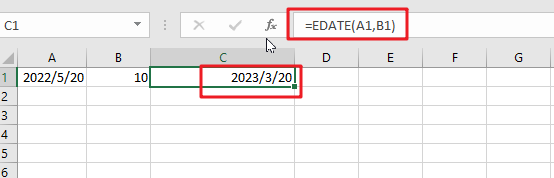The generic formula is:

`=EDATE(Sart_date, months)`
• `Start_date`: the starting date on which you want to add months
• `Months`: the month that you wish to add

Return Value:

The function will return the date that is the indicated number of months after the `start_date`. If you enter a negative number of months, it’ll count back from the end date.

When you enter the MONTH function, Excel looks at the date in the cell that you specify as the `start_date` argument. It then adds the number of months that you entered as the second argument to that date. If you enter a negative number for the second argument, Excel subtracts that number of months from the `start_date`.

To add a number of months is placed at B2 and add months to the date in cell A2. The formula for this would be:

`=EDATE(A1,B1)`Or simply enter the number you want in your formula, and it will be replaced with that value.

`=EDATE(A1,10)`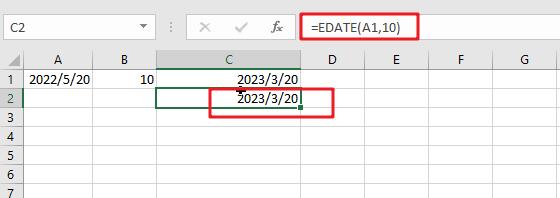### Explanation

This will add 10 months to the date in cell A1. The function will return the date that is the indicated number of months after the start_date. If you enter a negative number of months, it’ll count back from the end date.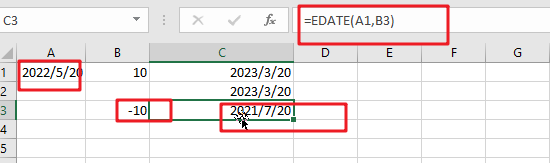You can also format the date in a certain way you want by right-clicking on it and then choosing ‘Format Cells’. In this pipping format, dialog select one date format type you want to display results. Then, press the Apply button to save changes made after filling out fields with proper data values following desired specifications, including today’s date if necessary!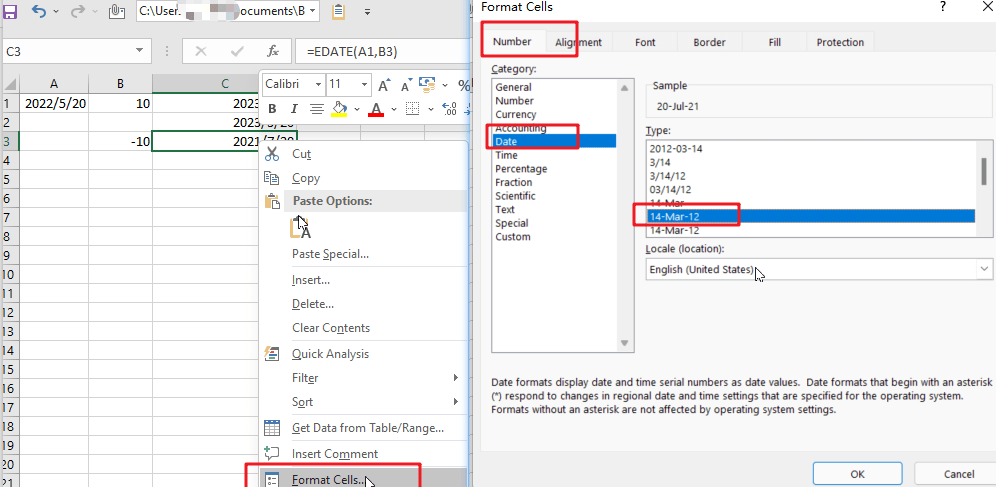The EDATE function is a great way to find out your next date. Simply enter any valid date and then select how many months away from now you want the new one! It’s really easy; just feed it with an amount less than or equal to the number of desired days between these two points-and voila.

Note:

Excel stores data as sequential serial numbers, which can be used in calculations. For instance, if you want to find out what day of the week it was on January 1st, 1900, then simply enter “serial#” into your formula bar and press Enter! The result will show that this date falls exactly 120 years ago – having been achieved by entering 44219 days after 1900 (which would make sense considering we’re talking about a Gregorian calendar).

## Method2: Add Months to Date using Date Function

The generic formula is:

`=DATE(YEAR(date),MONTH(date))+months,DAY(date)))`

### Arguments

• `Date`: The starting date on which you want to add months.
• Months: Months are important when adding or subtracting a certain number of months from/to dates. They add the indicated amount, depending on whether it’s positive and negative values respectively; for example, -1 means one month less than what you want (in other words: ago).
• YEAR(date): The year component of the date in Date.
• MONTH(date): The month component of the date in Date.
• `DAY(date)`: The day component of the date in Date.

### Return Value:

A date is the indicated number of months after the start_date. If you enter a negative number of months, it’ll count back from the end date.

### How This Formula Work

To add months to the date in cell A1, and a number of added is placed at B1. The formula for this would be as follows:

`=DATE(YEAR(A1),MONTH(A1)+B1,DAY(A1))`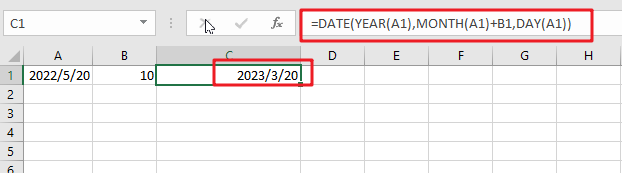With the Year, Month, and Day functions, you can easily create your date from any combination of these three values. With the Date function, you can create dates based on any year and month of your choice.

### Related Functions

• Excel DAY function
The Excel DAY function returns a day of a date (from 1 to 31).The DAY function is a build-in function in Microsoft Excel and it is categorized as a DATE and TIME Function.The syntax of the DAY function is as below:= DAY (date_value)…
• Excel EDATE function
TThe Excel EDATE function returns the serial number that represents the date that is a specified number of months before or after a specified date.The syntax of the EDATE function is as below:=EDATE (start_date, months)…
• Excel DATE function
The Excel DATE function returns the serial number for a date.The syntax of the DATE function is as below:= DATE (year, month, day)…
• Excel MONTH Function
The Excel MONTH function returns the month of a date represented by a serial number. And the month is an integer number from 1 to 12.The syntax of the MONTH function is as below:=MONTH (serial_number)…
• Excel YEAR function
The Excel YEAR function returns a four-digit year from a given date value, the year is returned as an integer ranging from 1900 to 9999. The syntax of the YEAR function is as below:=YEAR (serial_number)…

## How to Create a Summary Count by Month with COUNTIFS in Excel

This post will guide you how to create a summary count by month with COUNTIFS function in Excel 2013/2016 or Excel office 365. You can use the COUNTIF function to count cells with a given criteria in excel. this guide will show you the simple formula used to create a summary count by month with COUNTIFS function and the EDATE function with two criteria in Excel.

## Summary Count by Month with COUNTIFS

Assuming you have a list of data in range B1:B6 that contain date values, and you want to get a total count per month in your summary table(D1:D3) in your worksheet, and you can use a formula based on the COUNTIFS function to generate a total count per month. Like this:

``=COUNTIFS(\$B\$1:\$B\$6,">="&D1, \$B\$1:\$B\$6,"<"&EDATE(D1,1)) ``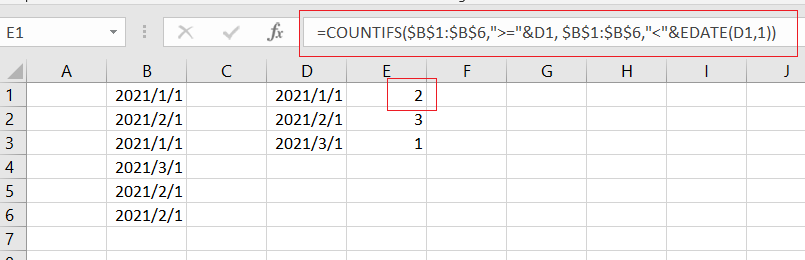Let’s See How This Formula works:

The COUNTIFS function can be used to count the number of cells that meet multiple conditions or criteria. And the Cell D1 is a date for the first of each month in 2021. And if you want to generate a total count per month, and you need to provide criteria that will filter all the date values that appear in each month in range B1:B6.

You still need to build criteria that is a second date can be created with the EDATE function. It means that dates must be greater than or equal to the data in cell D1 or less than the data in D1 plus one month (get it with EDATE function).

### Related Functions

• Excel COUNTIFS function
The Excel COUNTIFS function returns the count of cells in a range that meet one or more criteria. The syntax of the COUNTIFS function is as below:= COUNTIFS(criteria_range1, criteria1, [criteria_range2, criteria2]…)…
• Excel COUNTIF function
The Excel COUNTIF function will count the number of cells in a range that meet a given criteria. This function can be used to count the different kinds of cells with number, date, text values, blank, non-blanks, or containing specific characters.etc.= COUNTIF (range, criteria)…
• Excel EDATE function
TThe Excel EDATE function returns the serial number that represents the date that is a specified number of months before or after a specified date.The syntax of the EDATE function is as below:=EDATE (start_date, months)…

## How to Add / Subtract Days, Months and Years to Date

This post will guide you how to add or subtract a given number of days to a date in excel. How to add or subtract weeks to a date using excel formula. How to add or subtract a certain number of months to a date using excel formula. How to add or subtracts a given number of years to a date using an excel formula.  And how to add or subtract a combination of years, months and days to a date.

Assuming that you have a list of date in your worksheets, and you want to perform some arithmetic operations (such as, addition or subtraction) to calculate different time units, such as, you want to add or subtract a number of days to or from a date by using a formula to adjust the date according to your needs.

## Add / subtract days to date

If you have a list of date in a range or worksheet, and you want to add or subtract a certain number of days to the date in the range, you can refer to the following general formula:

`=Date + days`

Or

`=Date - days`

For example, to add 100 days to a date in Cell B1, then you can write the following formula in Cell C1:

=B1 +100

To subtract 100 days from a date in Cell B1, then you can write the following formula in Cell D1

=B1-100

If you want to add or subtract 100 days to a date in Cell b1, then you can use the TODAY function to build the following formula:

=Today() +100

Or

=Today() – 100

Let’s see the below screenshot to see the returned result by the above formulas: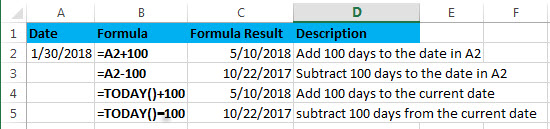## Add / subtract weeks to date

Assuming that you want to add or subtract a given number of weeks to a certain date in A2, then you can refer to the following generic formula:

`=Date +N Weeks * 7  `

Or

`=Date – N weeks*7`

You need to multiply the number of weeks by 7 to get the total number of days, then you can perform arithmetic operations in the same time unit (days).

Let’s see the below examples:

Supposing that you want to add or subtract 5 weeks to a date in A2, you can refer to the following excel formulas:

=A2 + 5*7

=A2 – 5*7

=TODAY() + 5*7

=TODAY() – 5*7

Let’s see the returned results by the above formula from the below screenshot: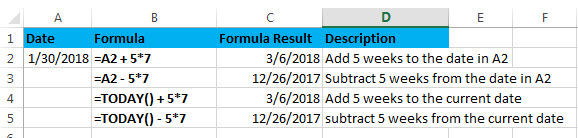## Add / subtract months to date

If you want to add or subtract a given number of whole months to a date, you can use the EDATE function to create a generic formula as follows:

`=EDATE(start_date, months)`

Start_date – the start date

Months – the number of months that you want to add or subtract

If you want to subtract months, you just need to enter a negative number as the months argument in the EDATE function.

Assuming that you want to add or subtract 3 months to the date in A2, you can use the following formula:

=EDATE(A2,3)

=EDATE(A2,-3)

=EDATE(TODAY(),3)

=EDATE(TODAY(),-3)

Let’s see the last result for above formulas: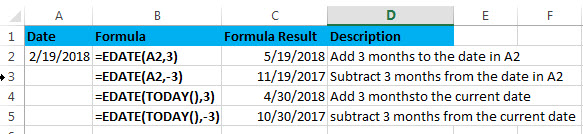## Add / subtract years to date

If you want to add or subtract a given number of years to a date, you can create an excel formula based on the DATE function, the YEAR function, the MONTH function and the DAY function.

The generic formula is as follows:

To add years to a date in excel:

`=DATE(YEAR(date)+ years, MONTH(date),DAY(date))`

To subtract years to a date in excel:

`=DATE(YEAR(date)+ years, MONTH(date),DAY(date))`

Assuming that you want to add or subtract 2 years to a date in A2, you can use the following formulas:

=DATE(YEAR(A2)+ 2, MONTH(A2),DAY(A2))

or

=DATE(YEAR(A2)- 2, MONTH(A2),DAY(A2))

Let’s see how this formula works:

=YEAR(A2)

Result: 2018

The YEAR function returns the year component of a date in A2.

=MONTH(A2)

Result: 2

The MONTH function returns the month portion of a date in A2.

=DAY(A2)

Result: 19

The DAY function returns the day of component of a date in A2.

Let’s see the following screenshot to show the result for above DATE formulas: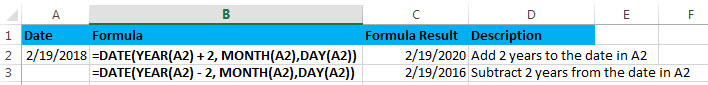## Add / subtract a combination of days, months and years

If you want to add or subtract a combination of days, months and years to a date in a single formula, you can also use a combination of the DATE function, the YEAR function, the MONTH function and the DAY function.

To add days, months and years in excel:

`=DATE(YEAR(start_date) + years, MONTH(start_date) + months, DAY(start_date) + days)`

To subtract days, months and years

`=DATE(YEAR(start_date) - years, MONTH(start_date) - months, DAY(start_date) - days)`

Assuming that you want to add or subtract 2 years, 3 months and 100 days to or from a date in cell A2, you can use the following formulas:

=DATE(YEAR(A2) + 2, MONTH(A2) + 3, DAY(A2) + 100)

Or

=DATE(YEAR(A2) – 2, MONTH(A2) – 3, DAY(A2) – 100)

Then enter the above formula into Cell C2 or C3, then let’s see the returned results: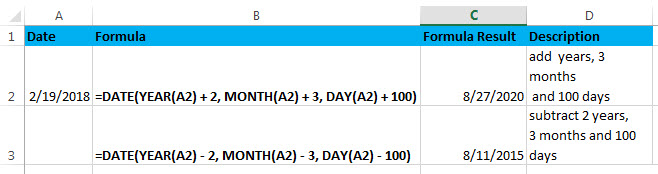### Related Formulas

• Convert date to month and year only in excel
If you want to convert the date to month and year only, you can use “yyyymm” format code within the TEXT function in excel, so you can write down the below TEXT formula:=TEXT(date,”yyyymm”)
• Convert date to month and day only in excel
If you want to convert the date (mm/dd/yyyy) to month and day only, you can use “mm dd” format code within the TEXT function in excel, so you can write down the below TEXT formula: =TEXT(date,”mm dd”)
• Convet Text Date dd/mm/yy to mm/dd/yyyy as Date Format
If you want to convert dates in the text format to the date format as mm/dd/yyyy, you can use a combination of the DATE function, the VALUE function, the RIGHT function, the MID function, and the LEFT function to create a new excel formula…

### Related Functions

• Excel DAY function
The Excel DAY function returns a day of a date (from 1 to 31).The DAY function is a build-in function in Microsoft Excel and it is categorized as a DATE and TIME Function.The syntax of the DAY function is as below:= DAY (date_value)…
• Excel EDATE function
TThe Excel EDATE function returns the serial number that represents the date that is a specified number of months before or after a specified date.The syntax of the EDATE function is as below:=EDATE (start_date, months)…
• Excel DATE function
The Excel DATE function returns the serial number for a date.The syntax of the DATE function is as below:= DATE (year, month, day)…
• Excel TODAY function
The Excel TODAY function returns the serial number of the current date. So you can get the current system date from the TODAY function. The syntax of the TODAY function is as below:=TODAY()…
• Excel MONTH Function
The Excel MONTH function returns the month of a date represented by a serial number. And the month is an integer number from 1 to 12.The syntax of the MONTH function is as below:=MONTH (serial_number)…

## Excel EDATE Function

This post will guide you how to use Excel EDATE function with syntax and examples in Microsoft excel.

## Description

The Excel EDATE function returns the serial number that represents the date that is a specified number of months before or after a specified date. You can use the EDATE function to calculate the expiration dates or maturity dates or due dates that fall on the same day of the month as the date of issue. or you can add a specified number of months to a date.

The EDATE function is a build-in function in Microsoft Excel and it is categorized as a  DATE and TIME Function.

The EDATE function is available in Excel 2016, Excel 2013, Excel 2010, Excel 2007, Excel 2003, Excel XP, Excel 2000, Excel 2011 for Mac.

## Syntax

The syntax of the EDATE function is as below:

`=EDATE (start_date, months)`

Where the EDATE function arguments are:

• Start_date -This is a required argument. Adate that represents the start date.
• months – This is a required argument. the number of months before or after start_date. if you provide a positive number for months, it represents the future date; if it is a negative number, then it represents a past date.

Notes:

• Microsoft Excel stores dates as a serial number, it indicated the number of days since January 1,1900. and the date January 1,1900 is serial number 1.
• if start_date is not a valid date, the EDATE function will return the #VALUE! error.
• if months is not an integer, it is truncated.

## Examples

The below examples will show you how to use Excel EDATE Function to return the serial number.
1# set a positive number for months argument in the EDATE function

`=EDATE(B1,2)`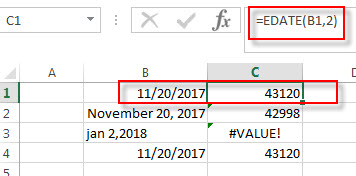2# set a negative number for months argument in the EDATE function, using the following formula:

`=EDATE(B2,-2)`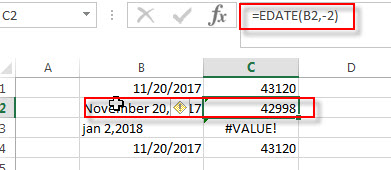3# set a invalid date for start_date argument in the EDATE function, type the following formula:

`=EDATE(B3,2)`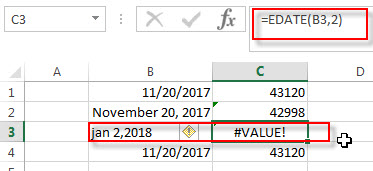4# set a value that is not an integer for months arguments, using the following formula:

`=EDATE(B4,2)`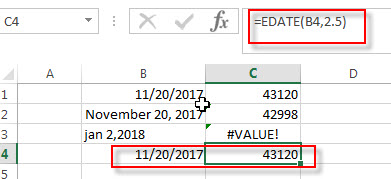### More Excel EDATE Function Examples

• Conditional Formatting date with red Amber or Green
How to highlight the date with red if the cell dates is past now(). How to highlight the date with amber if the cell date is past now but within the next 6 months from now(). How to highlight the date with green if the cell date is more than 6 months from now…